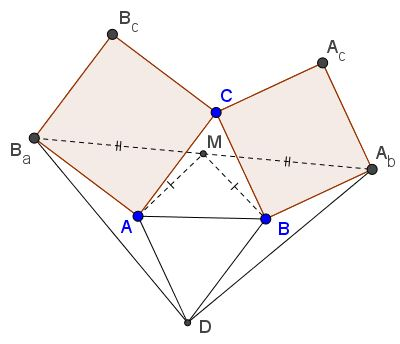# On Bottema's ShouldersWhat Is This About? A Mathematical Droodle

Put differently

Created with GeoGebra

ExplanationCopyright © 1996-2018 Alexander Bogomolny

### On Bottema's ShouldersConsider a configuration of two squares $ACB_{c}B_{a}\,$ and $BCA_{c}A_{b}\,$ with a common vertex $C.\,$ Bottema's theorem states that the midpoint $M\,$ of the segment $A_{b}B_{a}\,$ is independent of $C.\,$ Professor W. McWorter has observed another property of the configuration: if $D\,$ is the point that completes the parallelogram $ADBC,\,$ then $\Delta B_{a}DA_{b}\,$ is isosceles and angle $D\,$ is right: $\angle B_{a}DA_{b} = 90^{\circ}.$

The theorem is easily proved with complex numbers but also has a simple synthetic proof.

Continuing with the proof of Bottema's theorem, we assume that the vertices $A,\,$ $B,\,$ and $C\,$ are represented by the complex numbers $\alpha,\,$ $\beta,\,$ and $\gamma,\,$ respectively. We found there that

\begin{align} B_{a}&= \alpha + (\gamma - \alpha )\cdot i,\\ A_{b}&= \beta + (\gamma - \beta )\cdot (-i),\\ M&= (\alpha + \beta )/2 + (\beta - \alpha )\cdot i/2, \end{align}

where $i^2 = -1,\,$ as usual. We then obviously have

$i(A_{b} - M) = -i(B_{a} - M).$

Denoting the point $M + i(A_{b} - M)\,$ by $D,$

\begin{align} D&= M + i(A_{b} - M)\\ &= M + (-\gamma + (\alpha + \beta )/2 + i(\alpha - \beta )/2)\\ &= (\alpha + \beta ) - \gamma \\ &= \alpha + (\beta - \gamma ).\\ \end{align}

The latter tells us that $ADBC\,$ is a parallelogram.

The synthetic proofs starts with the observation that in triangles $AB_{a}D\,$ and $BDA_{b},$

\begin{align} AB_{a}&= BD\;\text{and}\\ AD&= BA_{b}. \end{align}

The angles between the equal sides are also equal, since both are found to be

$360^{\circ} - 90^{\circ} - \angle A - \angle B,$

the latter being the angles of $\Delta ABC\,$ at vertices $A\,$ and $B,\,$ respectively.

By Bottema's theorem, the clockwise rotation around M through $90^{\circ},\,$ maps $B\,$ on $A.\,$ Since $BA_{b}\,$ is perpendicular to $BC\,$ which, in turn, is parallel to $AD,\,$ the same rotation maps $BA_{b}\,$ on $AD\,$ and, similarly, $BD\,$ on $AB_{a}.\,$ It follows that the third sides of the triangles $AB_{a}D\,$ and $BDA_{b}\,$ are also mapped on each other. Thus the angle between them is $90^{\circ},\,$ as required.### Bottema's TheoremCopyright © 1996-2018 Alexander Bogomolny

 65243261